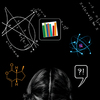# Thanks! You'll be called typically within 24 hours by the tutor or company you contacted.

Featured By### Arezu F.

#### Education

I have a M.Sc. in Biomechanical Engineering from McGill University. B.Sc. in Systems Engineering, Department of Intelligent Interaction technology, Graduate School of Systems and Information Engineering, University of Tsukuba, Ibaraki, Japan. In my undergrad and graduate studies, I took a range of math courses as a student and a TA.

#### Experience

Highly Experienced in Teaching different Algebra courses, Calculus, Pre-Calculus, Differential Equations and Statistics. List of Concordia Courses I Can Teach: MATH 200: FUNDAMENTAL CONCEPTS-ALGEBRA MATH 201: ELEMENTARY FUNCTIONS MATH 202: COLLEGE ALGEBRA MATH 203: DIFFERENTIAL&INTEGRAL CAL I MATH 204: VECTORS AND MATRICES MATH 205: DIFFERENTIAL&INTEGRAL CAL II MATH 206: ALGEBRA AND FUNCTIONS MATH 208: FUNDAMENTAL MATHEMATICS I MATH 209: FUNDAMENTAL MATHEMATICS II MATH 370: ORDINARY DIFFERENTIAL EQUAT. MATH 473: PARTIAL DIFFERENTIAL EQUATIONS MAST 214: CALCULUS AND LINEAR ALGEBRA MAST 330: DIFFERENTIAL EQUATIONS McGill Courses: MATH 112 FUNDAMENTAL OF MATHEMATICS MATH 122 CALCULUS FOR MANAGEMENT MATH 263 Ordinary Differential Equations for Engineers. MATH 140 Calculus 1. MATH 141 Calculus 2. MATH 139 Calculus 1 with Precalculus. MATH 133 Linear Algebra and Geometry MATH 111 Lecture Mathematics for Education Students. MATH 123 Lecture Linear Algebra and Probability. MATH 123 Lecture Linear Algebra and Probability. MATH 133 Lecture Linear Algebra and Geometry. MATH 133 Lecture Linear Algebra and Geometry. MATH 140 Lecture Calculus 1. MATH 141 Lecture Calculus 2. MATH 151 Lecture Calculus B. MATH 203 Lecture Principles of Statistics 1. MATH 204 Lecture Principles of Statistics 2. MATH 222 Lecture Calculus 3. MATH 223 Lecture Linear Algebra. MATH 236 Lecture Algebra 2. MATH 262 Lecture Intermediate Calculus. MATH 263 Lecture Ordinary Differential Equations for Engineers. MATH 264 Lecture Advanced Calculus for Engineers. MATH 314 Lecture Advanced Calculus. MATH 315 Lecture Ordinary Differential Equations.

#### Availability

Weekdays after 9AM, Weekends after 10AM

#### Can Meet

Up to 15 minutes away for no additional charge

#### Hobbies

I am part of Hit the Books, a group of superior Graduate Degree holders and PhD Candidates in diverse fields. We are proud that our team in Science, Engineering, Languages, and Art are among the Top University Candidates or Graduates in their respective fields. Highly experienced in tutoring, we are confidently able to help you with all your educational needs. We offer various educational services with the highest possible quality and at the most competitive prices in Montreal area. After all, we know how to Hit the Books! We Want You to Know too! For More Information, Please Contact "Hit the Books": info@hitthebooks.ca or (514) 699-2632, (514) 699-2699 www.hitthebooks.ca